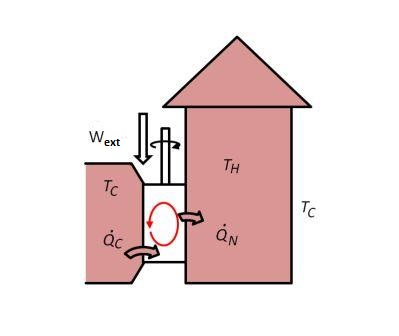# On a cold night, a house is losing heat at a rate of 15 ''kW'' . A reversible heat pump...

## Question:

On a cold night, a house is losing heat at a rate of 15 kW . A reversible heat pump maintains the house at {eq}20^{\circ}C {/eq} while the outside temperature is {eq}0^{\circ}C {/eq}.

Determine the heating cost for the night (8 hours). Assume the price of electricity to be 15 cents/kWh.

Also, determine the heating cost if resistance heating was used instead. Assume the price of electricity to be cents/kWh.## Heat Pumps:

• Heat pumps are mechanical devices used for space heating applications by receiving external electrical power.
• Coefficient of performance (COP) is an important parameter used to measure the performance of the heat pumps and refrigerators.

{eq}\textbf {Given:} {/eq}

• Rate of heat supplied to the house, {eq}\dot Q_H=15\ \text {kW} {/eq}
• Temperature of the house, {eq}T_H=20^\circ =293\ \text {K} {/eq}
• Surrounding temperature, {eq}T_C=0^\circ =273\ \text {K} {/eq}

Find the COP of the pump.

{eq}COP=\dfrac{T_H}{T_H-T_C}\\ =\dfrac{293}{293-273}\\ 14.65 {/eq}

Find the power supplied to the heat pump.

{eq}COP=\dfrac{\dot Q_1}{\dot W}\\ 14.65=\dfrac{15}{\dot W}\\ \dot W=1.024\space kW {/eq}

Heating cost for 8 hours.

{eq}=1.024\times 8\times 0.15\\ =1.229 {/eq}

Thus, the heating cost for the night is {eq}\bf \color {navy} {\text {1.229}}. {/eq} dollars.

Assuming the price of electricity as 15 cents per kWh, find the heating cost if resistance heating is used.

{eq}=15\times 8\times 0.15\\ =18 {/eq} .

Thus, if resistance heating is used, the heating cost for the night is {eq}\bf \color {navy} {\text {18.00}} {/eq} dollars.Types of Heating & Cooling Systems

from

Chapter 10 / Lesson 9
2.7K

You can choose from a number of different heating and cooling systems to make your home comfortable to live in. Watch this video to learn about the advantages and disadvantages of forced air, radiant heat, baseboard heat and geothermal systems.